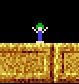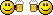###Author Topic: Point inside polygon  (Read 5996 times)

####doimus

• Dr. Type
••• Posts: 284##### Point inside polygon
« on: 2011-Oct-09 »
Here's some code that finds whether a particular point is inside a polygon.
Actual routine is not mine, I've found the C code online (http://www.visibone.com/inpoly/inpoly.c) and translated it into GLB.

It's great for implementing various regions, zones, collision or whatever else into your programs.
Code is pure math, it has nothing to do with graphical polygons (no acceleration) but it supports all kinds of mad polygonal shapes.

Code: (glbasic) [Select]
`SYSTEMPOINTER TRUE//create polygonLOCAL poly[]DIM poly[RND(3)+3]FOR i = 0 TO BOUNDS(poly[], 0) - 1 poly[i] = RND(640) poly[i] = RND(480)NEXT// main loopWHILE TRUE LOCAL mx,my,mba,mbb MOUSESTATE mx, my, mba, mbb drawPoly(poly[]) IF inPoly(poly[], mx, my) PRINT "Inside!",0,0 ENDIF SHOWSCREENWENDFUNCTION inPoly: poly[], tx, ty LOCAL newx, newy, oldx, oldy LOCAL x1, y1, x2, y2 LOCAL inside LOCAL npoints = BOUNDS(poly[], 0) IF npoints < 3 THEN RETURN 0 oldx = poly[npoints - 1] oldy = poly[npoints - 1] FOR i = 0 TO npoints - 1 newx = poly[i] newy = poly[i] IF newx > oldx x1 = oldx x2 = newx y1 = oldy y2 = newy ELSE x1 = newx x2 = oldx y1 = newy y2 = oldy ENDIF IF ((newx < tx) = (tx <= oldx) AND (ty-y1)*(x2-x1) < (y2-y1)*(tx-x1)) inside = NOT inside ENDIF oldx = newx oldy = newy NEXT RETURN inside ENDFUNCTIONFUNCTION drawPoly: poly[] LOCAL npoints = BOUNDS(poly[], 0) FOR i = 0 TO npoints-2 DRAWLINE poly[i],poly[i], poly[i+1],poly[i+1], RGB(200,200,200) NEXT DRAWLINE poly[-1],poly[-1], poly,poly, RGB(200,200,200) ENDFUNCTION`
« Last Edit: 2014-May-03 by Moru »

####AMateus

• Mr. Drawsprite
•• Posts: 61##### Re: Point inside polygon
« Reply #1 on: 2011-Oct-09 »
NiceThank you for sharing.

####matchy

• Prof. Inline
••• Posts: 1545##### Re: Point inside polygon
« Reply #2 on: 2011-Oct-10 »
Cool. Looks faster than the code I'd converted from http://www.vb-helper.com/howto_find_angles.html

Code: (glbasic) [Select]
`TYPE _vertex pts[] // polygon x y z width height off_x off_yENDTYPEFUNCTION PointInPolygon: ply AS _vertex, point AS _vertex LOCAL c, corners, total_angle LOCAL ver AS _vertex corners = BOUNDS(ply.pts[], 0) - 1 total_angle = GetAngleProduct(ply.pts[corners], ply.pts[corners], point.x, point.y, ply.pts, ply.pts) FOR c = 0 TO corners - 1 ver.x = ply.pts[c] + ply.x ver.y = ply.pts[c] + ply.y ver.off_x = point.x ver.off_y = point.y ver.width = ply.pts[c + 1] + ply.x ver.height = ply.pts[c + 1] + ply.y     total_angle = total_angle + GetAngleProduct(ver.x, ver.y, ver.off_x, ver.off_y, ver.width, ver.height) NEXT RETURN ABS(INTEGER(total_angle))ENDFUNCTIONFUNCTION GetAngleProduct: Ax,Ay,Bx,By,Cx,Cy LOCAL dot_product, cross_product dot_product = DotProduct(Ax, Ay, Bx, By, Cx, Cy) cross_product = CrossProductLength(Ax, Ay, Bx, By, Cx, Cy) RETURN ATan2(cross_product, dot_product)ENDFUNCTIONFUNCTION ATan2: opp,adj LOCAL angle LOCAL iPI = 3.14159265358979323846 IF ABS(adj) < 0.0001     angle = iPI / 2 ELSE     angle = ABS(ATAN(opp, adj)) ENDIF IF adj < 0     angle  = iPI - angle ENDIF IF opp < 0     angle = -angle ENDIF RETURN angleENDFUNCTIONFUNCTION CrossProductLength: Ax,Ay,Bx,By,Cx,Cy LOCAL BAx=Ax-Bx LOCAL BAy=Ay-By LOCAL BCx=Cx-Bx LOCAL BCy=Cy-By RETURN (BAx*BCy) - (BAy*BCx)ENDFUNCTIONFUNCTION DotProduct: Ax,Ay,Bx,By,Cx,Cy LOCAL BAx= Ax - Bx LOCAL BAy= Ay - By LOCAL BCx= Cx - Bx LOCAL BCy= Cy - By RETURN (BAx*BCx) + (BAy*BCy)ENDFUNCTION`

####Wampus

• Prof. Inline
••• Posts: 1004##### Re: Point inside polygon
« Reply #3 on: 2012-Apr-19 »
Cheers me dears. I was just about to write a routine to do this myself. Now I won't have to.####xbee

• Mc. Print
•• Posts: 10##### Re: Point inside polygon
« Reply #4 on: 2013-Nov-11 »
Perfect. Just what I was looking forMany thanks.

####mentalthink

• Prof. Inline
••• Posts: 3372
• Integrated Brain##### Re: Point inside polygon
« Reply #5 on: 2013-Nov-12 »
Thanks -domius very interesting the code, perhaps for void make a for over  a huge mesh can works fine?¿...
Thanks I think I use your code in my simple 3D Editor...